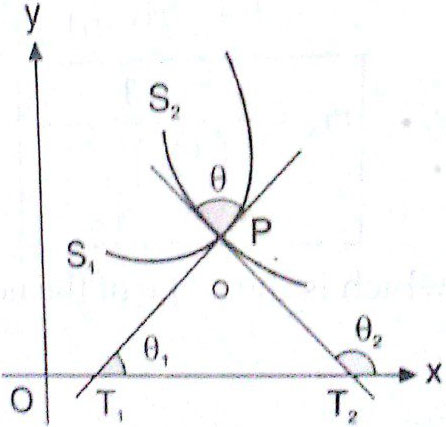# The angle of intersection between  and  is  at a point, where abscissa is positive, then  equals ? Option 1) 1 Option 2) 2 Option 3) 3 Option 4) 4

H Himanshu

As we have learned

Angle of intersection of two curves -

The angle of intersection of two curves is the angle subtended between the tangents at their point of intersection.Let  m1  &  m2 are two slope of tangents at intersection point of two curves then

- whereinwhere  is angle between two curves tangents.

and  x+y =1

point of intersection are (0,1) and (1,0)  we need to find angle at (1,0)

at ( 1,0) will be infinite , i.e tangent will be vertical

makes  with x-axis so with a vertical line it will  makeOption 1)

1

Option 2)

2

Option 3)

3

Option 4)

4

Exams
Articles
Questions